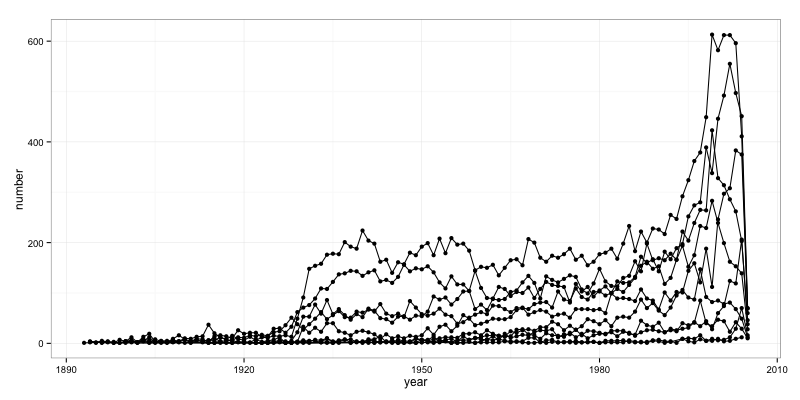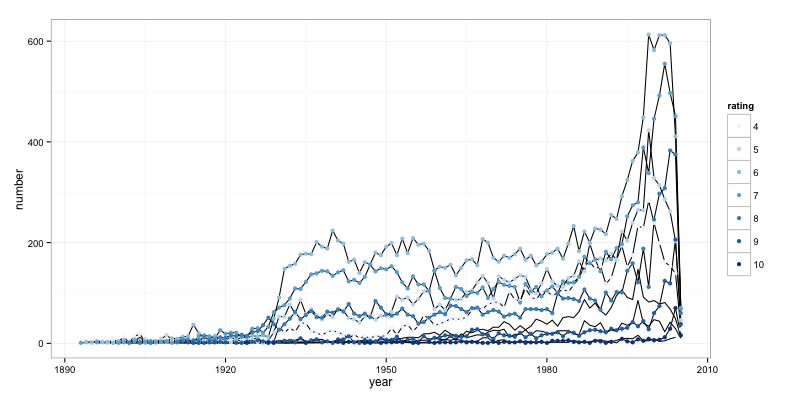# if else condition in ggplot to add an extra layer

say I want to plot two layers in ggplot, one containing points and another one containing lines if a certain criteria is fulfilled.

The code without the criteria could look like this:

``````library("ggplot2")

# Summarise number of movie ratings by year of movie
mry <- do.call(rbind, by(movies, round(movies\$rating), function(df) {
nums <- tapply(df\$length, df\$year, length)
data.frame(rating=round(df\$rating), year = as.numeric(names(nums)), number=as.vector(nums))
}))

p <- ggplot(mry, aes(x=year, y=number, group=rating))

p +
geom_point()+
geom_line()
``````

now the condition for plotting the points and not only the lines would be, that an object called tmp.data does not equal the expression "no value".

``````tmp.data<-c(1,2,3) # in this case the condition is fulfilled

# attempt to plot the two layers including the condition in the plotting function
p+
if(tmp.data!="no value"){ geom_point()+}
geom_line()
``````

fails....

``````Error: unexpected '}' in:
"p+
if(tmp.data!="no value"){ geom_point()+}"
``````

geom_line() geom_line:
stat_identity:
position_identity: (width = NULL, height = NULL)

• Why not just change the order: `p + geom_line() + if(tmp.data!="no value"){ geom_point()}` Apr 7, 2014 at 14:40
• @shadow. this works only if my second layer is the last argument in the function. if I add further arguments like: `+ theme(panel.background = element_rect(fill = "lightskyblue2"))` it does not work anymore Apr 7, 2014 at 14:54

This was done using ggplot2 2.1.0. I think you can do exactly what the OP wished, just by switching the parenthesis so that they encompass the entire `if` statement.

Here is an example that add a horizontal line depending on if `Swtich` is `T` or `F`. First, where the condition is `TRUE`

``````library(ggplot2)

df<-data.frame(x=1:10,y=11:20)
Switch=T

ggplot(df,aes(x,y))+
{if(Switch)geom_hline(yintercept=15)}+
geom_point()
``````Now, the same thing but the condition is `FALSE`

``````df<-data.frame(x=1:10,y=11:20)
Switch=F

ggplot(df,aes(x,y))+
{if(Switch)geom_hline(yintercept=15)}+
geom_point()
``````• it seems that you cannot use the `+` inside of the `{`, so if you want to conditionally add multiple things to a ggplot, I believe you need to add another `if` statement (inside of its own `{`) for each object you add to the plot. Jan 8, 2018 at 15:54
• You cannot use `+` inside the `{`, but you can put everything into a `list` if you have multiple steps. `{if(Switch)list(geom_hline(yintercept=15), geom_hline(yintercept = 13))}` Jun 24, 2020 at 19:07
• @StephenK in a million years, I would never have intuited that this was the right way to execute multiple steps inside `{}`, but thankfully I was able to find your comment! Feb 13 at 2:46

What you are seeing is a syntax error. The most robust way I can think of is:

``````tmp.data<-c(1,2,3)
if(tmp.data!="no value") {
p = p + geom_point()
}
p + geom_line()
``````

So you compose the object `p` in a sequence, only adding `geom_point()` when the if statements yields `TRUE`.

• this solution doesn't work if there trying to facet_wrap. I get the error: Error in seq.default(min, max, by = by) : 'from' must be a finite number
– val
Oct 16, 2018 at 21:49

Following the ggplot2 book, you can create a function which returns a list. Any NULL components will be ignored.

``````library(ggplot2)
library(ggplot2movies)

# Summarise number of movie ratings by year of movie
mry <- do.call(rbind, by(movies, round(movies\$rating), function(df) {
nums <- tapply(df\$length, df\$year, length)
data.frame(rating=round(df\$rating), year = as.numeric(names(nums)), number=as.vector(nums))
}))

# create function to add points conditionally
# If the list contains any NULL elements, they’re ignored.
my_plot <- function(point = FALSE){
list(
geom_line(),
if (point)
geom_point()
)

}

p <- ggplot(mry, aes(x=year, y=number, group=rating))
p + my_plot()
````````````p + my_plot(point = TRUE)
``````Created on 2020-02-25 by the reprex package (v0.3.0)

``````library(ggplot2)

# Summarise number of movie ratings by year of movie
mry <- do.call(rbind, by(movies, round(movies\$rating), function(df) {
nums <- tapply(df\$length, df\$year, length)
data.frame(rating=round(df\$rating), year = as.numeric(names(nums)), number=as.vector(nums))
}))

tmp.data<-c(1,2,3) # in this case the condition is fulfilled

p <- ggplot(mry, aes(x=year, y=number, group=rating))

# this won't "loop through" the data points but it's what you asked for
if (tmp.data!="no value") {
p <- p + geom_point() + geom_line()
} else {
p <- p + geom_line()
}
p
``````but perhaps this is more like what you really want?

``````mry\$rating <- factor(mry\$rating)
p <- ggplot(mry, aes(x=year, y=number, group=rating))
p <- p + geom_line()
p <- p + geom_point(data=mry[!(mry\$rating %in% tmp.data),],
aes(x=year, y=number, group=rating, color=rating), size=2)
p <- p + scale_color_brewer()
p
``````An alternative example using `iris` dataset creating a plot with conditional components, some with more than one line, and with multiple switches.

``````library("ggplot2")

p <- ggplot(iris,
aes(x=Sepal.Length,
y=Sepal.Width,
colour=Species))

# set plot switches to turn on or off various components
include_points = TRUE
include_lines = FALSE
include_density = TRUE
include_facet = TRUE

p +
list(
if(include_points){geom_point()},
if(include_lines){geom_line()},
if(include_facet){

# multi-line conditional elements can be included as a list
list(
facet_grid(rows = "Species"),
labs(subtitle = "This plot has been faceted, and colour has been removed"),
aes(colour = NULL)
)

},
if(include_density){geom_density2d()}) +

# additional elements common to all possible conditional combinations
# can be added as well
theme_minimal() +
labs(title = "Plot with conditional elements")
``````

With the conditions as follows:

``````include_points = TRUE
include_lines = FALSE
include_density = TRUE
include_facet = TRUE
``````

The following plot is generated:

Faceted plot with density and points

Setting `include_facet = FALSE` the following plot is generated:

Coloured plot with density - no facets